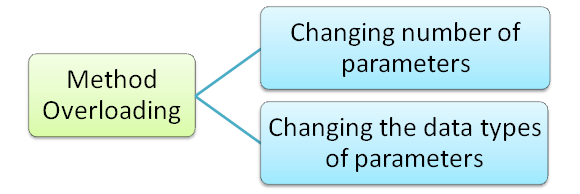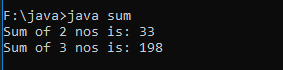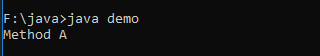A method or a function is an integral and most important part of any object oriented language. A function can be defined as: a block of statements which perform a specific task. Methods describe the behaviour of an object. Java Method Overloading is a form of polymorphism.

Method Overloading is a feature that allows a class to have a function with more than one definition. It means that methods with the same name but the argument lists are different. Either the number of arguments are different or the type of arguments are different but the function name is the same. These functions may however be used for different purposes.

When an overloaded function is invoked (or called), the compiler differentiates the methods with the same name from each other by two things:

1. The number of arguments in each method.
2. The data types of each argument.

The version of the method which matches in both the above respects gets invoked. Hence when we overload any method we can overload it in two different ways. These are:

##Changing the number of arguments/parameters

Let us take an example:

```class sum
{
// Function 1
{
System.out.println("Sum of 2 nos is: " + (a+b));
}

// Function 2
void add(int a, int b, int c)
{
System.out.println("Sum of 3 nos is: " + (a+b+c));
}

public static void main(String args [])
{
sum s = new sum();

// Calling
}
}```

### Output:In the above example, we have overloaded the function add two times. In the first function, we have two arguments both of integer types. In the second definition, we have the same function with three arguments of type integers. At run time, the compiler will call the desired function which matches the prototype and executes the block of statements written in that function.

## Changing the data type of arguments/parameters

Let us take another example:

``` class SumNum
{
// Function 1
{
System.out.println("Sum when both numbers are Integers: " + (a+b));
}

// Function 2
{
System.out.println("Sum when one number is Integer and other is Float: " + (a+b));
}

// Function 3
{
System.out.println("Sum when one number is Float and other is Integer: " + (a+b));
}

public static void main(String args [])
{
SumNum s = new SumNum();

// Calling
}
}```

### Output:In the above example, we have overloaded the function add three times. In the first function, we have two arguments both of integer types. In the second definition, we have the same function with arguments of different data types i.e. first argument is of integer data type and second is of float data type.

In the third definition, we have the same function with first argument is of float data type and second is of integer data type. At run time, the compiler will call the desired function which matches the prototype and will execute the block of statements written in that function.

When a data type of smaller size is promoted to the data type of a bigger size then it is referred to as type promotion. For example: byte data type is promoted to short, short to int, int to long, long to float and float to double.

### Example

``` class demo
{
void show(int a, double b)
{
System.out.println("Method A");
}
void show(int a, int b)
{
System.out.println("Method B");
}
public static void main(String args[])
{
demo obj = new demo();
obj.show(100, 20.6f);
}
}```

### Output:Here a call was made using float data type but there was no function with that prototype. So, float was automatically promoted to double and the function was called. If this would not have happened, an error would have appeared on the screen.